# 数据缩放：标准化和归一化

## 数据缩放简介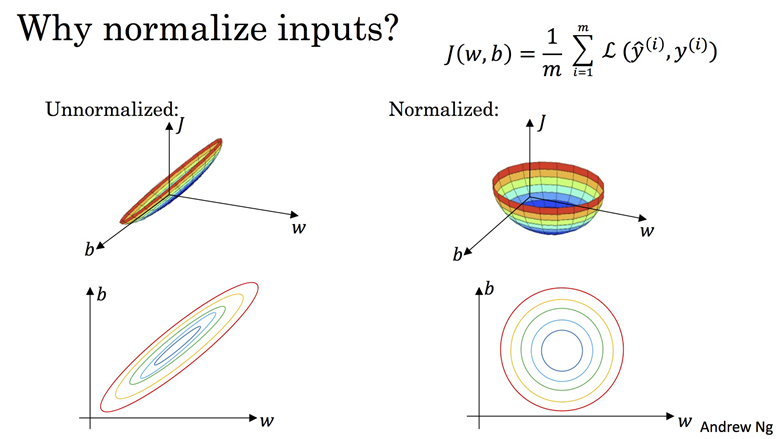• 基于距离的算法（Distance-Based Algorithms）：在基于距离进行优化的算法中，需要进行特征缩放，比如K近邻K-MeansSVMPCA等。因为这些算法是基于数据点的特征值计算它们的距离，距离越小则两者越相似。
• 基于树的算法（Tree-Based Algorithms）：基于树的算法对特征（features）的数值范围并不敏感，比如决策树。决策树仅基于单个feature拆分节点，并不受其它feature的影响。
• 线性判别分析朴素贝叶斯等算法：这两个算法处理了特征数量级差异大的问题，因此不需要进行特征缩放。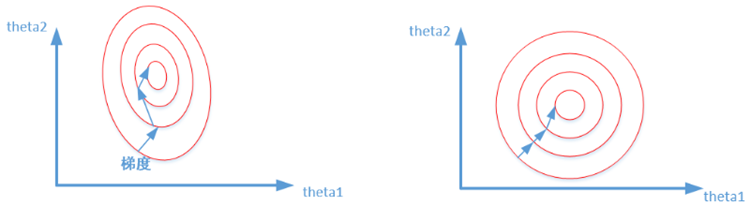### 指标一致化

• 对原始数据取倒数（下单频率与下单次数）
• 定义不同指标中数值上限，然后依次减去每个指标中的原始数据（比如死亡率与生存率）

### 无量纲化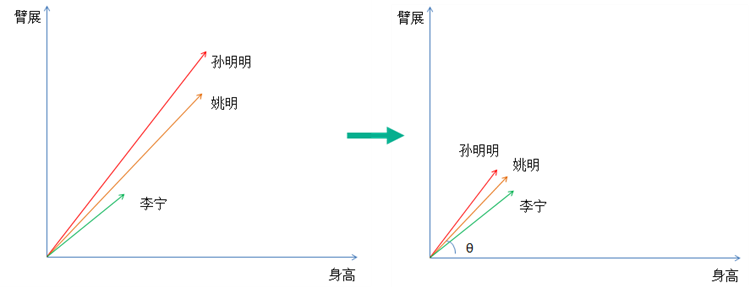#### Min-Max归一化（Min-Max Normalization）

Min-Max归一化又称为极差法，最简单处理量纲问题的方法，它是将数据集中某一列数值缩放到0和1之间。 它的计算方法是：

$${x}’=\frac{x-x_{min}}{x_{max}-x_{min}}$$

#### 最大绝对值法（MaxAbs）

$${x}’=\frac{x}{x_{max}}$$

MaxAbs方法跟Max-Min用法类似，也是将数据落入一定区间，但该方法的数据区间为[-1,1]。MaxAbs也具有不破坏原有数据分布结构的特点，因此也可以用于稀疏数据。

#### 均值归一化（Mean Normalization）

$${x}’= \frac{x-\mu }{x_{max}-x_{min}}$$

#### log函数转化

Log函数也可用于归一化。结果落到[0,1]区间上，具体公式为：

$${x}’=\frac{\log x}{\log x_{max}}$$

#### atan函数转换

$${x}’= \frac{atan(x) * 2}{\pi}$$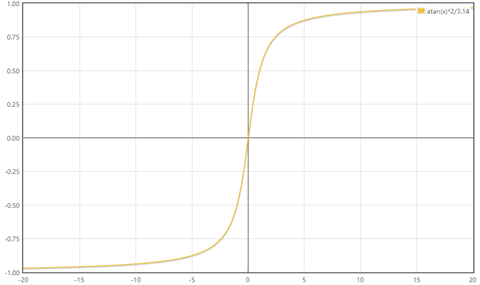#### Sigmoid函数转换

Sigmoid函数是一个具有S形曲线的函数，是良好的阈值函数，在(0, 0.5)处中心对称，在(0, 0.5)附近有比较大的斜率，而当数据趋向于正无穷和负无穷的时候，映射出来的值就会无限趋向于1和0。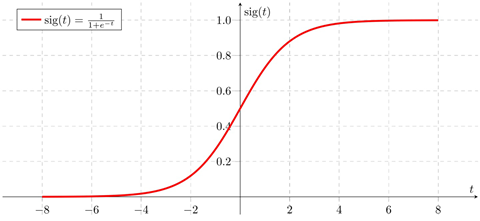$${x}’= \frac{1}{1+{e}^{-x}}$$

#### Decimal scaling

$$x^*=\frac{x}{10^j}$$

#### Z标准化：实现中心化和正态分布

z-score标准化也叫标准差标准化，代表的是分值偏离均值的程度，经过处理的数据符合标准正态分布，即均值为0，标准差为1。其转化函数为

$${x}’= \frac{x-\mu }{\sigma }$$

#### 修改型z-score标准化

$${x}’= \frac{x-x_{median} }{d}$$

d为所有样本数据的绝对偏差,其计算公式为：

$$d = \frac{1}{N}\sum_{1}^{n}|x_i – x_{median}|$$

#### RobustScaler

$${x}’ = \frac{x-x_{median}}{{IQR}}$$

RobustScaler 函数使用对异常值鲁棒的统计信息来缩放特征。这个标量去除中值，并根据分位数范围(默认为IQR即四分位数范围)对数据进行缩放。IQR是第1个四分位数(第25分位数)和第3个四分位数(第75分位数)之间的范围。通过计算训练集中样本的相关统计量，对每个特征分别进行定心和缩放。然后将中值和四分位范围存储起来，使用“变换”方法用于以后的数据。

### 标准化、归一化的区别

• 如果数据集小而稳定，可以选择归一化
• 如果数据集中含有噪声和异常值，可以选择标准化，标准化更加适合嘈杂的大数据集。

## Scikit-Learn中标准化和归一化方法

sklearn.preprocessing提供了许多方便的用于做数据预处理工具，在数据标准化方面，sklearn.preprocessing提供了几种scaler进行不同种类的数据标准化操作：

• StandardScaler
• MinMaxScaler
• MaxAbsScaler
• RobustScaler

• .fit(): 用于计算训练集train_x的均值、方差、最大值、最小值等训练集固有的属性。
• .transform(): 用于在fit()的基础上对指定的数据集（训练集、测试集、验证机）进行标准化、降维、归一化等变换。
• .fit_transform()：整合fit()和transform()，同时实现属性学习和变换，该函数仅仅为了简化操作。

### 标准步骤
# 1. 设置某种预处理方法
scaler = method()
# 2. 使用训练数据来获取训练集的固有属性
scaler.fit(x_train)
# 3. 应用到训练集、测试集和验证集
x_train_scaled = scaler.transform(x_train)
x_test_scaled = scaler.transform(x_test)
x_val_scaled = scaler.transform(x_val)

### 第一种简化方法
# 其中第1.2步可以简化为：
scaler = method().fit(X_train)

### 第二种简化方法
# 为了简化操作，可以将第以上操作简化为：
# 1. 设置某种预处理方法
scaler = method()
# 2. 使用训练数据来获取训练集的固有属性，并应用到训练集
x_train_scaled = scaler.fit_transform(x_train)
# 3. 应用到训练集、测试集和验证集
x_test_scaled = scaler.transform(x_test)
x_val_scaled = scaler.transform(x_val)

import pandas as pd
from sklearn.preprocessing import StandardScaler

ss = StandardScaler()
scale_features = ['r', 'f', 'm']
df[scale_features] = ss.fit_transform(df[scale_features])


cols=list(df)   # 可以改成自己需要的列的名字
for item in cols:
max_tmp = np.max(np.array(df[item]))
min_tmp = np.min(np.array(df[item]))
if (max_tmp != min_tmp):
df[item] = df[item].apply(lambda x: (x - min_tmp) / (max_tmp - min_tmp))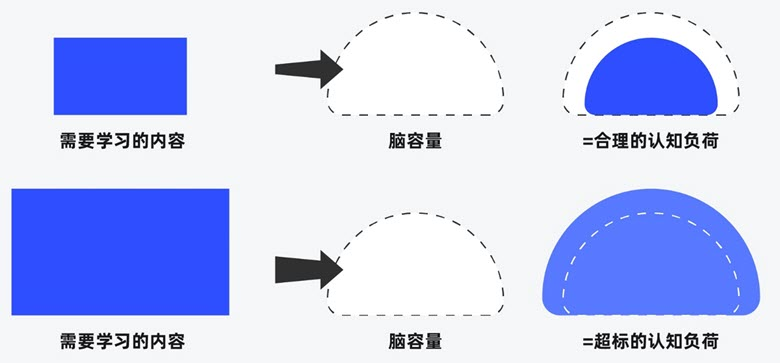##### 界面设计和游戏设计中的认知负荷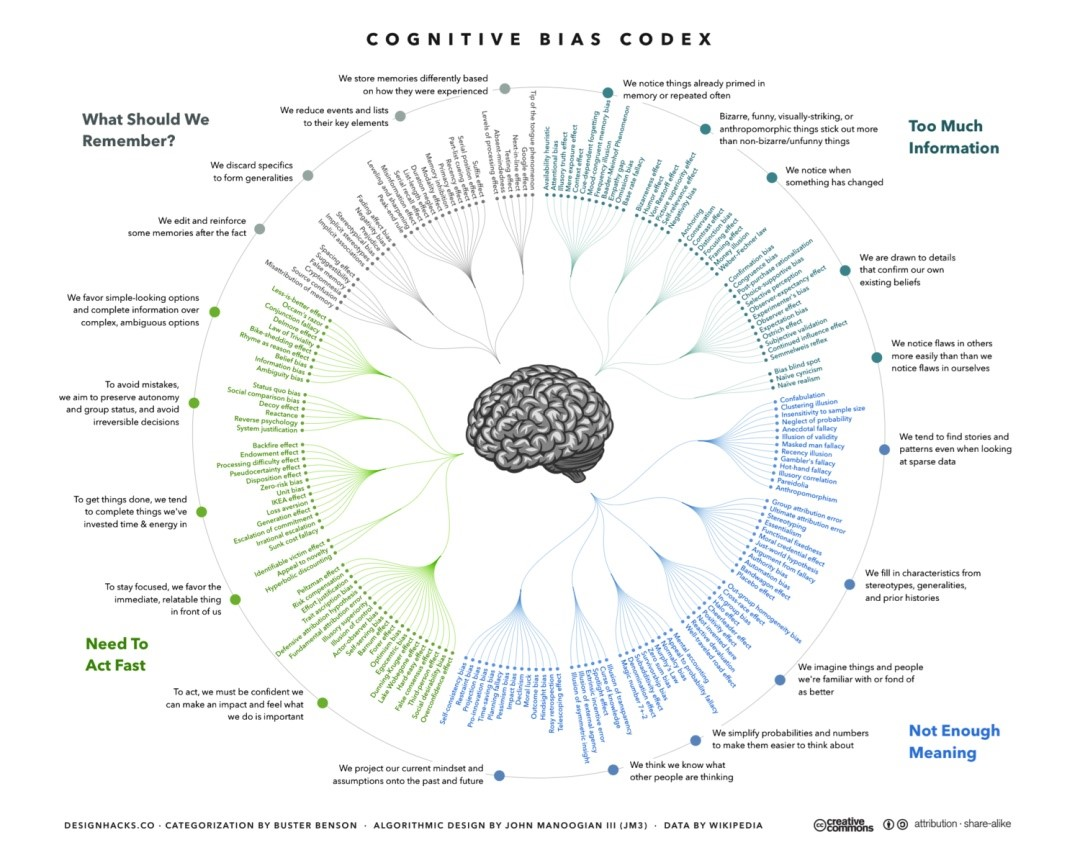##### 理解认知偏差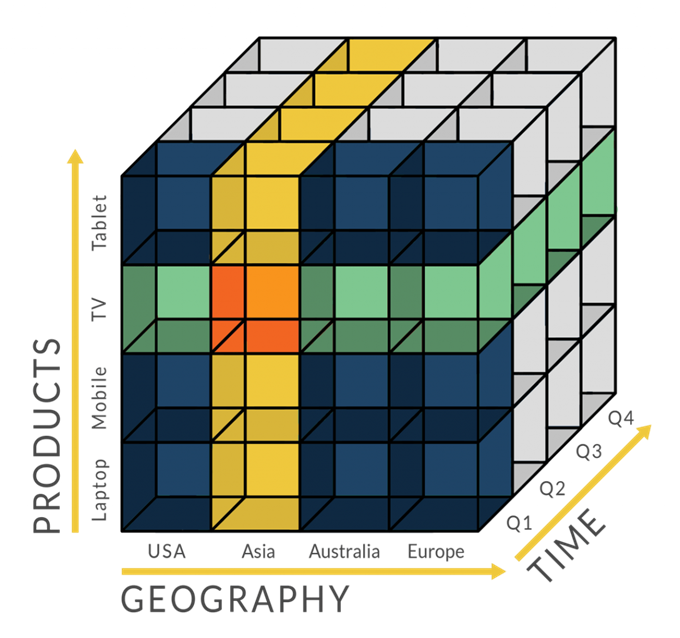## 2 Replies to “数据缩放：标准化和归一化”

1. 写得很好~~~，看了很多篇博文，感谢博主。。

2. 夏仔阳说道：

感谢博主~~~很适合复习